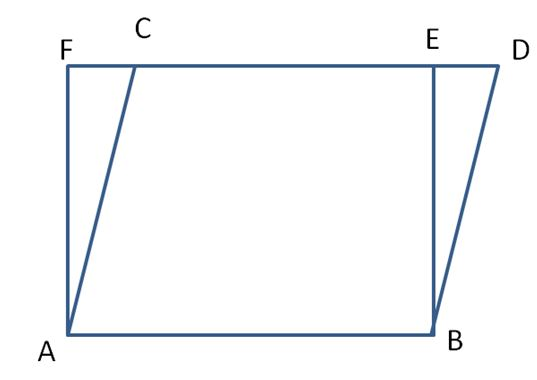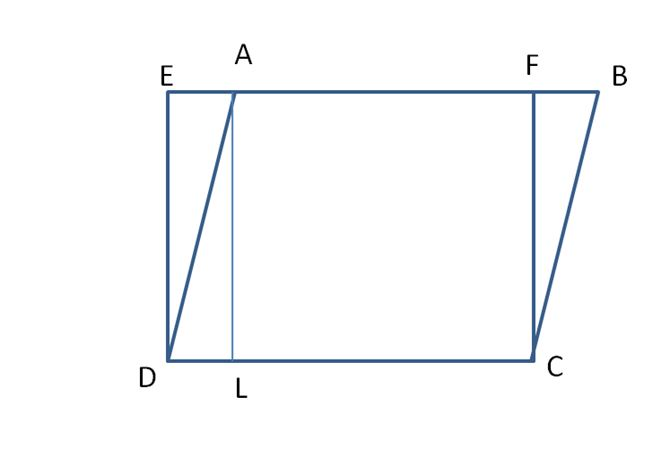# Parallelograms and Rectangles on Same Base and between Same Parallels – Theorem, Examples

When one parallelogram and a rectangle lies on the same base and between the same parallels then they measure equal area. Here we are providing the example questions, answers for Parallelograms, and Rectangles on Same Base and between the Same Parallels. We are also offering proof that shows parallelogram and rectangle on same between same parallels have the same area.

## Proof for Rectangles and Parallelograms on the Same Base have Equal Area

Below-mentioned is the steps for the parallelogram and rectangles having the same base and between the same parallels having equal area.

In the following figure, ABCD is a parallelogram and ABEF is the rectangle that lies on the same base AB and the same parallels AB and DF.Area of parallelogram ABCD = base x altitude

AF is the altitude of the parallelogram.

So, Parallelogram area = AB x AF.

Area of the rectangle ABEF = base x height

= AB x AF

Therefore, the area of the parallelogram ABCD = Area of the rectangle ABEF.

### Solved Examples for Parallelograms and Rectangles on Same Base and between Same Parallels

Example 1:

Parallelogram ABCD and rectangle ABFE have the same AB and the length and breadth of the rectangle are 15 cm and 9 cm. Find the area of the parallelogram.

Solution:

Given that,

Length of the rectangle l = 15 cm

The breadth of the rectangle b = 9 cm

Parallelogram ABCD and rectangle ABFE have the same AB.

So, area of the parallelogram = Area of the rectangle.

Area of the rectangle = length x breadth

= 15 x 9 = 135 cm²

Therefore, area of the parallelogram is 135 cm².

Example 2:

In the adjacent figure, ABCD is a parallelogram and EFCD is a rectangle. Also, AL ⊥ DC. Prove that(a) Area (ABCD) = Area (EFCD)

(b) Area (ABCD) = DC × AL

Solution:

(a)

Rectangle is CDEF.

Parallelogram is ABCD.

In the given figure, AL and DE are parallel and have the same length. So, DE = AL.

Area of ABCD = CD x AL

= CD x DE square units.

Area of EFCD = CD x DE

So, Area of EFCD = Area of ABCD

Hence proved.

(b)

As ABCD is a parallelogram.

Area of ABCD = base x altitude

The base is CD and the altitude is AL.

Therefore, Area of ABCD = CD x AL

Hence proved.

Example 3:

Parallelogram ABCD and rectangle ABFE have the same AB and the base and height of the parallelogram are 28 cm and 16 cm. Find the area of the rectangle.

Solution:

Given that,

Base of the parallelogram = 28 cm

Height of the parallelogram = 16 cm

Area of the parallelogram = base x height

= 28 x 16 = 448 cm²

We know, that when Parallelograms and Rectangles on Same Base and between Same Parallels, then the area of the parallelogram = area of the rectangle.

So, the area of the rectangle = 448 cm².